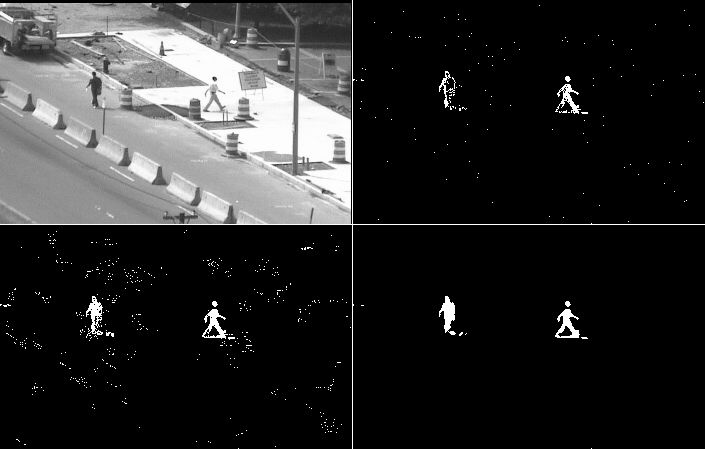Team: M.J. McHugh, J. Konrad, V. Saligrama, D. Castanon
Funding: Air Force Office of Sponsored Research (SBIR), College of Engineering Catalyst Award
Status: Completed (2007-2008)

Background: Identification of regions of interest in the field of view of a camera from the standpoint of occurring dynamics (movement, other changes), often called background subtraction,  is a core task in many computer vision and video analytics problems. To date the problem has been attacked from many angles and it seems that the algorithms implementing background subtraction are quite mature. Still, even the best algorithms today occasionally fail or deliver sub-par performance. Is it possible to further improve performance of background subtraction, i.e., reduce false positive and false negative rates?

Summary: We have revisited background subtraction from the standpoint of binary hypothesis testing. In particular, instead of a simple background probability test PB(I) > t, where PB(I) is the probability that intensity I is in the background and t is a threshold, we apply a hypothesis test PB(I)/PF(I) > t, where PF(I) is the probability that intensity is in the foreground (moving pixel). Since PF(I) cannot be estimated from the same-position pixels in past frames (unless motion of the moving object is known), we assume spatial ergodicity of intensity patterns and use spatial, instead of temporal, history to compute the foreground probability PF(I). We also incorporate prior probability into the binary hypothesis test via a Markov random field model that accounts for spatial background label coherence.

Results: This combination of foreground and prior Markov models leads to an improvement in the detection accuracy as shown below. These particular results are based on non-parametric kernel model (Parzen window) for both PB (temporally over 80 frames) and PF (spatially over previous-iteration foreground pixels within 7×7 neighborhood). Note a significant reduction of both false positives and negatives.

 Frame from original video                                                              PB(I) thresholdingPB(I)/PF(I) thresholding                                                   PB(I)/PF(I) with Markov model

Below are shown video sequences with results for four methods. Each video shows the results in a 2×2 grid as follows:

 PB(I) thresholding PB(I)/PF(I) thresholding PB(I) thresholding with Markov model PB(I)/PF(I) thresholding with Markov mod

Results for the same threshold t used in all 4 methods:

Synthetic sequence: natural objects undergo synthetic motion against natural background

Masspike: motor traffic on I-90 in Boston and its overpass

Sidewalk:  pedestrain traffic on Commonwealth Avenue in Boston

Note the excessive false positives for the non-Markov methods (top row); Markov models remove the vast majority of false detections and show superior performance. Improvements due to the introduction of foreground model are difficult to assess due to the clutter in non-Markov methods. It is very subtle in Markov-based methods (some holes in moving objects are filled in).

Lower threshold t for non-Markov methods (top row) – to equalize the number of false positives among all results:

Synthetic sequence: natural objects undergo synthetic motion against natural background

Masspike: motor traffic on I-90 in Boston and its overpass

Sidewalk:  pedestrain traffic on Commonwealth Avenue in Boston

Although improvements due to the introduction of the foreground model are subtle, a careful inspection reveals a reduction of false negatives (holes in moving cars, pedestrians) while maintaining the same level of false positives. The Markov model again consistently outperforms non-Markov approaches.

Publications: